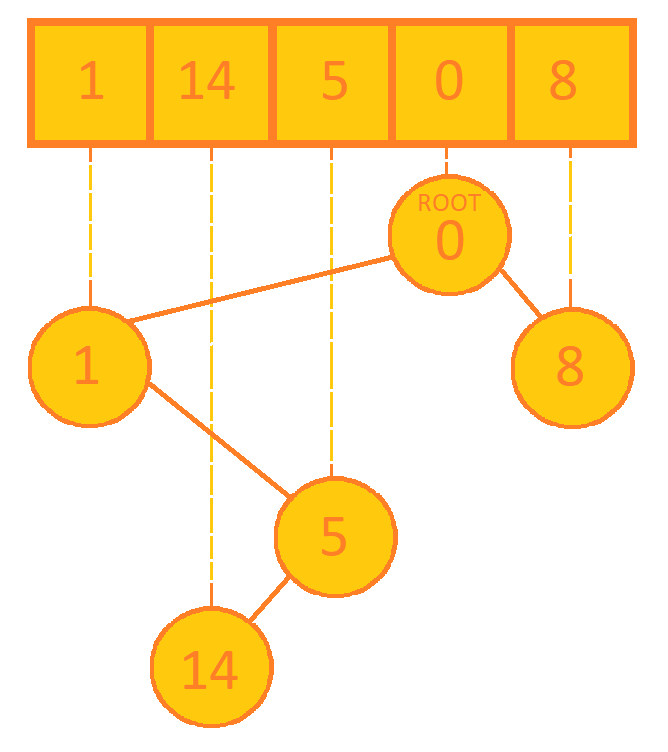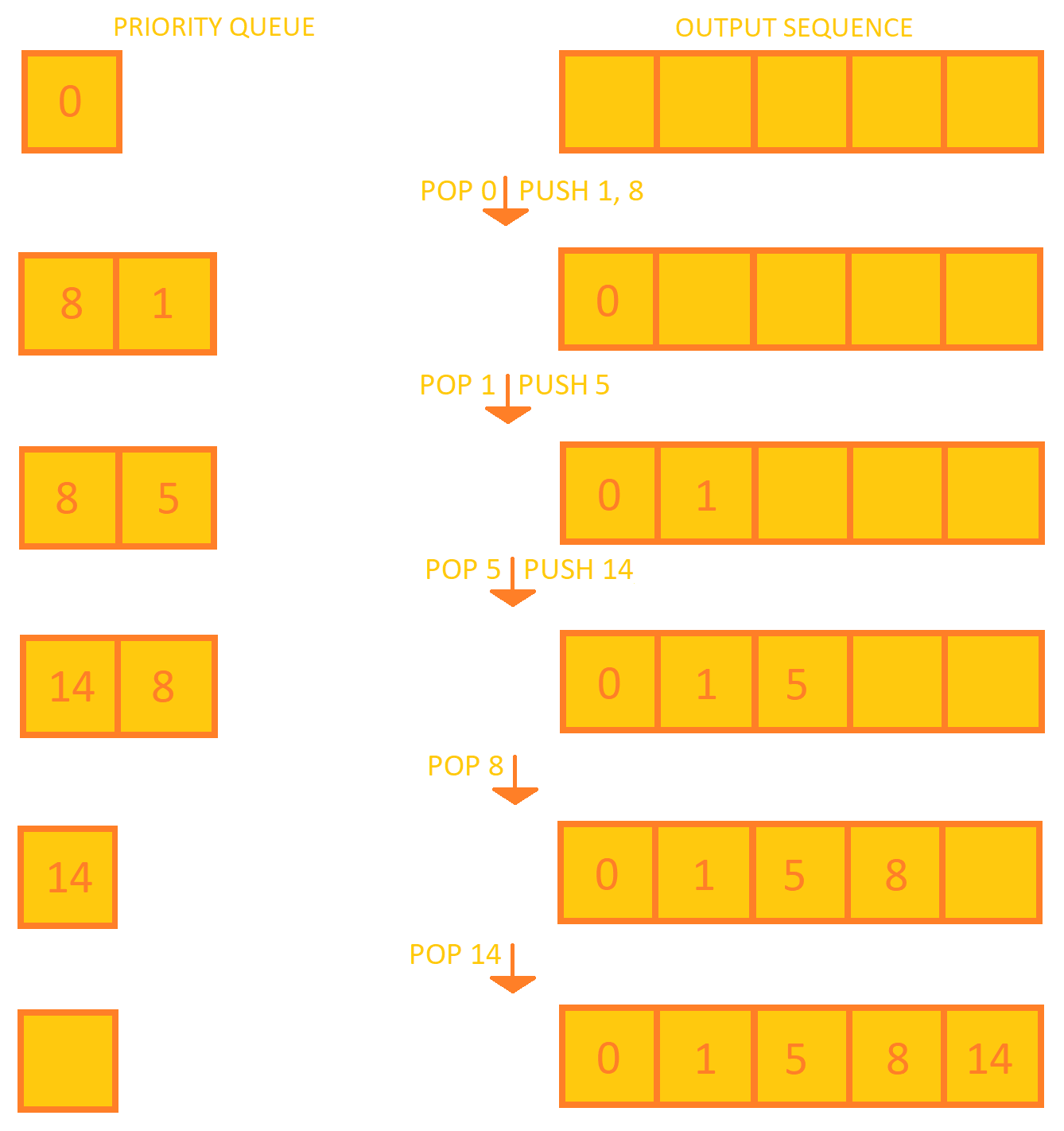×

Search anything:

# Cartesian tree sorting

#### Algorithms Sorting Algorithms cartesian tree priority queue queueIndian Technical Authorship Contest starts on 1st July 2023. Stay tuned.

Reading time: 30 minutes | Coding time: 20 minutes

Cartesian tree sorting, also called Levcopoulos Petersson algorithm is an adaptive sorting algorithm, i.e. it performs much better on a partially sorted data. It needs two data structures: a Cartesian tree and a priority queue. The algorithm here uses min-heap Cartesian tree to give a sorted sequence. We can also use max-heap Cartesian tree but that will give a sorted sequence in non-increasing order.

This algorithm performs better than just using a priority queue/heap because it saves lot of operations used to adjusting a heap done when pooping a top element. It basically maintains a priority queue of candidates, and at each step it removes the smallest element to put at the end of sequence while also performing pre-order traversal on Cartesian tree. Pre-order traversal is used since parent is always smaller than the children in the tree.

### Algorithm

1. Construct a Cartesian tree for given sequence.
2. Create a priority queue, initially having only the root of Cartesian tree.
3. Pop the minimum value x from the priority queue
4. Add x to the output sequence
5. Push the left child of x from Cartesian tree into priority queue.
6. Push the right child of x from Cartesian tree into priority queue.
7. If priority queue is not empty, goto step 3.

The Cartesian tree sorting for the sequence {1, 14, 5, 0, 8} will be:### Complexity

• Worst case time complexity: Θ(NlogN)
• Best case time complexity: O(N)
• Space complexity: O(N)

### Implementations

C++ 11

/*
* c++ 11 code to construct a Cartesian tree. The method cartesianTreeSort
* will sort the contents of the array.
*/

#include <iostream>
#include <vector>
#include <queue>

struct Node{
int value;
Node *left, *right;
Node *parent;

Node(){
value = 0;
parent = NULL;
left = NULL;
right = NULL;
}
};

// Used by priority queue
struct compare{
bool operator()(Node *left, Node *right){
return left->value > right->value;
}
};

class CartesianTree{
private:
// last pointer to keep track of last node added
Node *root, *last;

Node * findLowestNode(Node *node, int x){
if(node->value < x)
return node;
else if(node->parent != NULL)
return findLowestNode(node->parent, x);
else
return NULL;
}

public:
Node * getRoot(){
return root;
}

Node *new_node = new Node;
new_node->value = x;
if(root == NULL){
last = new_node;
root = new_node;
return;
}
Node *z = findLowestNode(last, x);
if(z == NULL){
new_node->left = root;
root->parent = new_node;
root = new_node;
}
else{
new_node->left = z->right;
z->right = new_node;
new_node->parent = z;
}
last = new_node;
}

CartesianTree(std::vector<int> ar){
root = NULL;
last = NULL;
// Call addNode function for each element of the array
for(auto x : ar){
}
}

void InorderTraversal(Node *node){
// To print inorder traversal of the tree
if(node == NULL)
return;
InorderTraversal(node->left);
std::cout << node->value << ' ';
InorderTraversal(node->right);
}

// Function to sort and store values in array
void cartesianTreeSort(std::vector<int> &sorted_ar){
// Initialize input array
sorted_ar.assign(0, 0);

// Initialize priority queue
std::priority_queue<Node *, std::vector<Node *>, compare> p_queue;
p_queue.push(root);
Node *temp = NULL;
while(!p_queue.empty()){
temp = p_queue.top();
p_queue.pop();
sorted_ar.push_back(temp->value);
if(temp->left){
p_queue.push(temp->left);
}
if(temp->right){
p_queue.push(temp->right);
}
}
}
};

int main(){
std::vector<int> ar = {1, 14, 5, 0, 8};

CartesianTree tree(ar);
std::cout << "Inorder Traversal\n";
tree.InorderTraversal(tree.getRoot());
std::cout << '\n';

std::vector<int> sorted;
tree.cartesianTreeSort(sorted);
std::cout << "Sorted array is\n";
for(auto x : sorted)
std::cout << x << ' ';
std::cout << '\n';
}

### Applications

• It is possible to quantify how much faster algorithm will run than O(nlogn) using a measurement called oscillation. Practically, the complexity is close to O(nlog(Osc)) where Osc is oscillation.
• Oscillation is small if the sequence is partially sorted, thus the algorithm performs faster with partially sorted sequences.